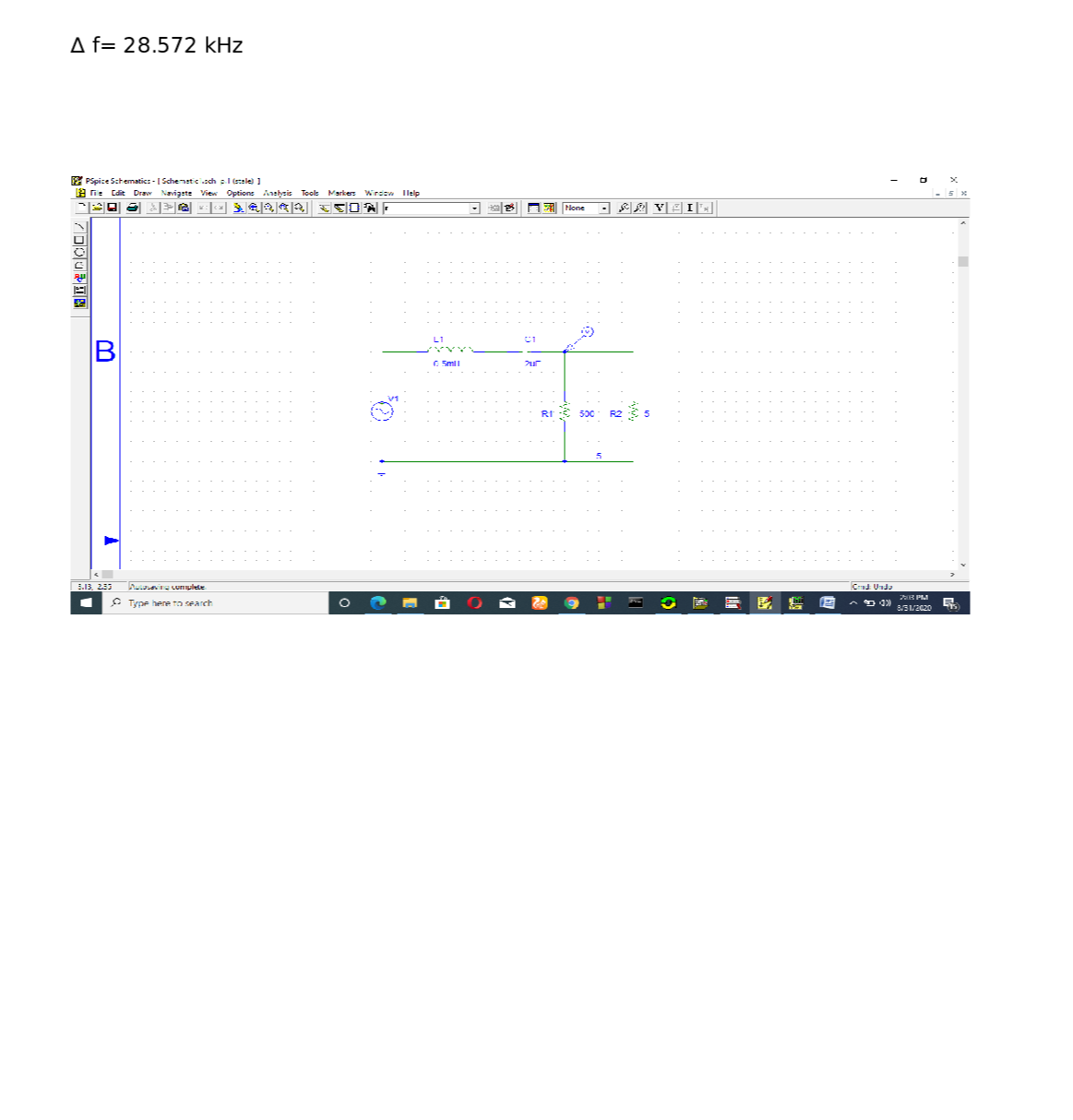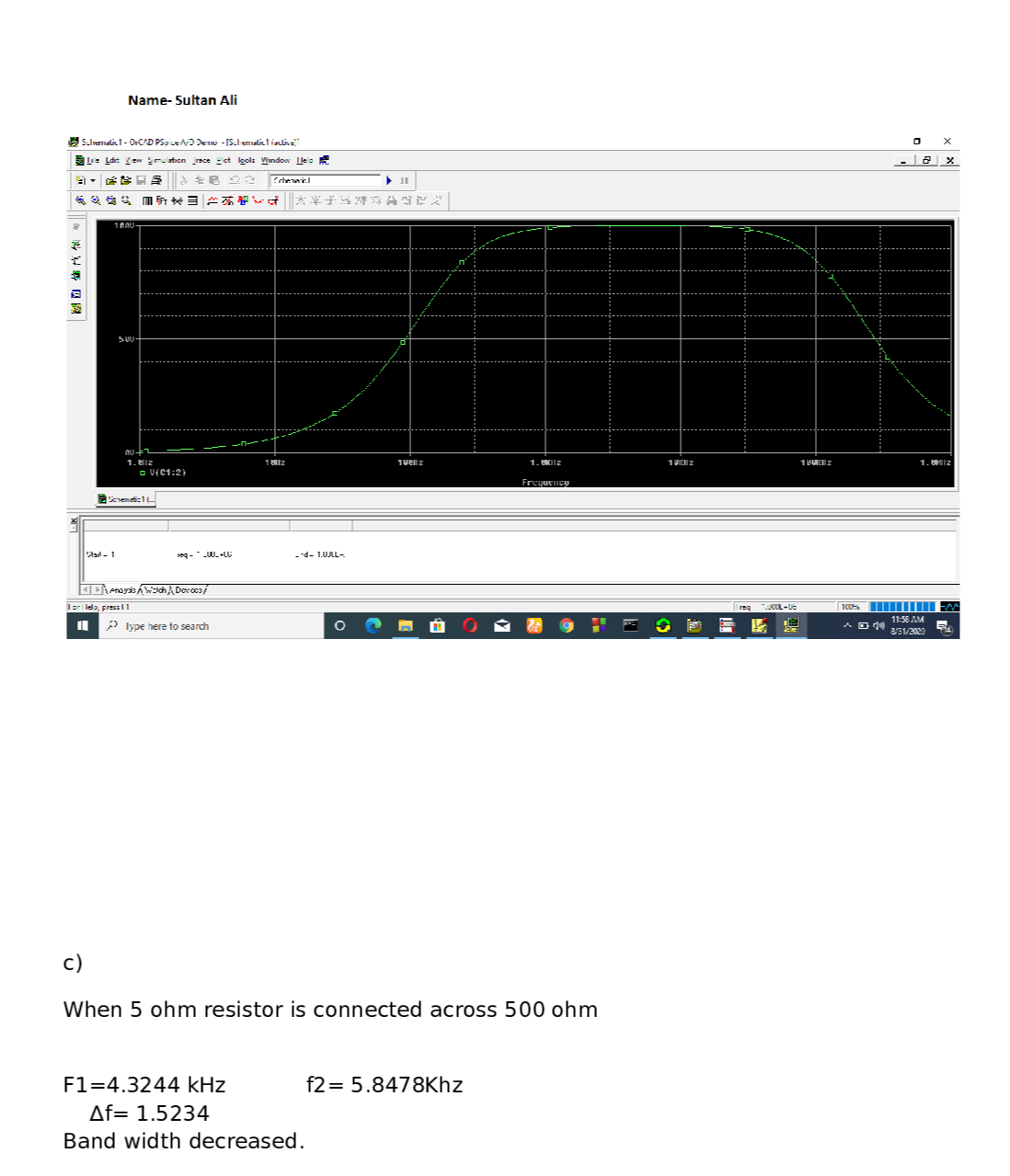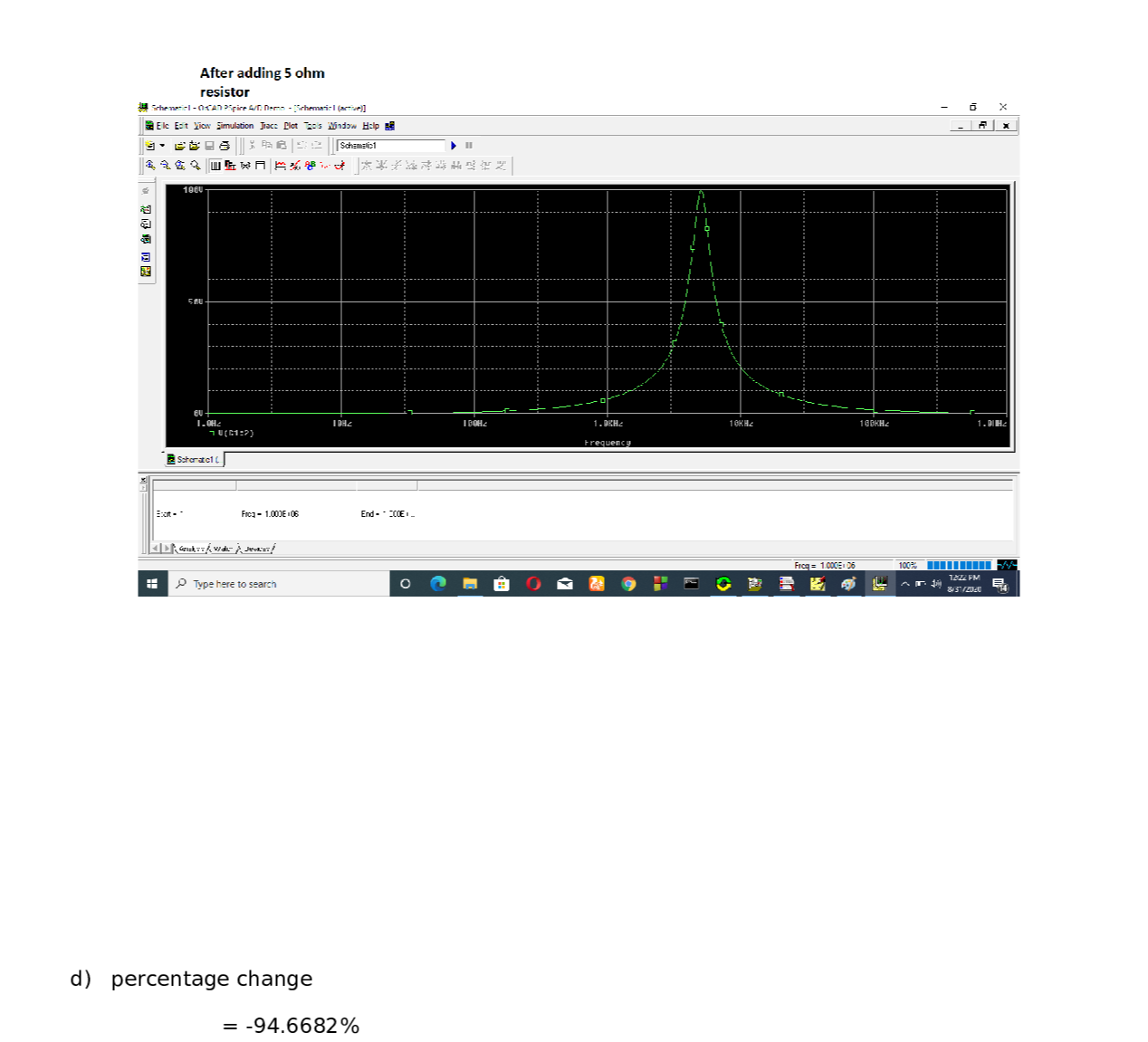Question

Data Science

Design a serier RLC bandpass filter with a center frequency of 5 KHz and a quality factor of 5. Use a 2 µF capacitor.

a- Calculate the value of R and L?

b- Use the P Spice to plot the output voltage versus frequency , for (1 <f<1 MHz), Vin =100 Vrms. Mark both cut - off frequencies on the plot and print it. Make sure that you print your name in the polt.

Wc2 =

then calculate Wc1=

C- A 5 Q resistor is connected across the output terminals of the filter (across R). Add this load to your filter and re simulate the program again. Print the new Vout versus frequency polt twith new cutt-off frequencies marked on the plot.

fc2 new (pspice)=

fc1 new (pspice)=

what is the effect of adding resistor in the band width?

d- Calculate the percentage change in the band width for the two different cases b&c.Verified### Question 41127Data Science

Given the start vertex s = a in the weighted directed graph below, use the following table to show the result of Dijkstra’s Algorithm (reference Section 8.4).(12 Points)

### Question 41126Data Science

Briefly describe the two different ways to find a path given the start vertex and the end vertex in a graph. (6 Points)

### Question 41125Data Science

Given a graph G=(V, E), what is its adjacent matrix and what is its incident matrix? (8Points)

### Question 41124Data Science

Assume that an array has n elements. Prove that the complexity of the binary search isO(log. n). (10 Points)

### Question 41123Data Science

Assume that an array has n elements. Prove that the complexity of the sequential search isO(n). (10 Points)

### Question 41122Data Science

Assume that the elements in the integer array a are in ascending order. Complete the recursive binary search function below that returns the index of the target value t: (10Points)

### Question 41121Data Science

a) What is a priority queue?A priority queue takes item's priority into account. When items in the queue are accessed, the item with the highest priority is accessed first.
. Answer the following questions: (12 Points)
b) What is a binary search tree?
c) What is a heap?

### Question 41120Data Science

3. Complete the integer queue class defined below: (12 Points)

### Question 41119Data Science

Complete the integer stack class defined below: (10 Points)

### Question 41118Data Science

1. Assume that f(n) = 2n² + 3n +1. Figure out M, N>0, when n>N, |f(n)|<M|n²l, i.e.f(n)=O(n³). (10 Points)

### Submit query